# Lesson 1: Overview of A/B Testing

A/B testing consists of choosing a metric, reviewing statistics, designing experiments, and analyzing results. A/B testing is a general control/experiment methodology used online to test out a new product or a feature. For example, two groups of users act on two versions of websites, their activities will be recorded, some metrics will be computed based on the activities, and the metrics will be used to evaluate the two versions. A variety of things can be tested, from some new features, additions to your UI, different look for you website. Examples:

• Amazon launching personalized recommendations increases the revenue

However, not for testing out new experiences. (changing features in a UI or on a website is not significant enough for a new experience?) For experience, two questions: what is your baseline and how long to wait the users adapt to the new experiences due to change a version and novelty effect. Or long-term problems vs. A/B testing is short term. Missing features.

# When can we use A/B testing?

• Could try specific products but can’t answer in general, ask users
• Too long to test repeat customers and don’t have data for referrals

## When A/B testing cannot be used, what other techniques?

Retrospective analysis with log data -> hypothesis -> design and randomize experiments for a perspective analysis -> A/B testing the hypothesis. User experience research, focus group, surveys, and human evaluation. It is difficult to use A/B testing for new experience.

## A/B testing has other names

agriculture, hypothesis testing (clinical trials), consistent response from your control and your experiment group, online A/B testing has lower resolution of users’ information, clinical trials have more detailed information of the participants.

# Overview of an example

• choose a metric

# Choose a metric

• Click through rate: # of clicks on one button or link / # of page views containing the button or link.

The difference is CTR cares about clicks and CTP cares about visitors. A visitor may click and view page multiple times. In general, a rate is used to measure the usability and a probability is used to measure the impact. For example, use rate to answer how often a user finds a specific button on a web page with many buttons; use probability to answer how many users progress to the next page. For CTR, engineers modify the website to capture a page view event and a click event. For CTP, need to further match each page view with all of the child clicks, so that you count, at most, one child click per page view (this is assuming each pager view comes from a different user). (match each user to a page view?)

# Review statistics (binomial distribution, confidence interval, hypothesis testing)

Review why binomial distribution converges to normal distribution as n increases and how to compute confidence interval for probability p

## Binomial distribution

Say we have arandom variable Xn follows a Binomial distribution, Xn ~ b(n, p). We can consider Xn = sum(Xi), where Xi follows Bernoulli distribution, Xi ~ b(1, p). The above picture summarizes some examples when you can or cannot use the binomial distribution.

## Confidence interval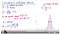As a binomial distribution converges to normal distribution when n is large, the formula of the margin of error uses the z score from N(0, 1).

This lesson shows how to estimate the confidence interval of the probability p of a binomial distribution: b(n, p). As n increases, a binomial distribution converges into a normal distribution. But how to estimate the parameters of the normal distribution given the binomial distribution. Here is an example, k~ b(n, p) -> N(np, np(1-p)) or p^ ~ b(n, p) -> N(p, p(1-p)/n), where k is the # of successes and p^ is the fraction of success out of n tries with a probability of success p.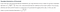The difference of computing the number of successes (k) and the fraction of successes (p)

Thus, given a sample (100 flips, 40 heads), the sample probability is x, which is 40/100 in this case. We have the following equation:

`P(|(x - p)/sqrt(p(1-p)/n)| < u(1-alpha/2)) > 1 - alphaAfter a few conversions...x - u(1-alpha/2)*sqrt(x(1 - x)/n) < p < x + u(1-alpha/2)*sqrt(x(1-x)/n)`

Recall that binomial distribution can also converge to poisson distribution when n is large and p is small. If np > 5 or n(1-p) > 5, then use normal distribution.

## Hypothesis testing

P(results due to chance | H0 is True) = p value

The video shows the hypothesis testing for two means of binomial distribution, similar to two means of normal distribution, using the pooled standard error. Not the hypothesis testing for one mean.

Review the hypothesis testing for two means and large sample

## Practical or Substantive significance

Hypothesis testing can test whether the result is statistically significant. It essentially tests the repeatability of the experiments, which guarantees the change observed in one experiment are repeatable in other experiments with the same set-up. But you also want to know whether the change is interesting from a business perspective. Practical significance means what size of change matters. As a statistician, we care about wha’s substantive in addition to being statistically significant. It will answer whether it is worth the change considering all the costs to make the change.

`For example,H0: p0 = p1, Ha: p0 <> p1The p value of this hypothesis testing is smaller than 0.05, so the difference is statistically significant. Then what is the difference, (p1 - p0)? Is it substantive?For online cases, if p1 - p0 > 2%, that is already a substantive significance. What if the metric is an absolute number, like the number of clicks or subscriptions? `

“So you want to size your experiment appropriately, such that the statistical significance bar is lower the practical significance bar.” ???

# Design

## Sample Size and Power trade-off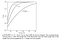From Design and Analysis of Experiments by Douglas Montgometry, Chapter 2

The above figure clearly shows that given an effect size (the difference of two means divided by the standard deviation), the power increases as the sample size increases. Given a sample size, the power increases as the effect size increases, that is, larger effect size is more easily to be detected. (But in the lesson, the effect size is 2%, the absolute difference of click-through probability ???).

From the lesson, “When we see something interesting, we want to make sure that we have enough power to conclude with high probability that the interesting result is, in fact, statistically significant.” By “interesting result”, it means the difference of two means or the effect size. So we need a probability to tell us the power to detect this difference. The p values shows whether the difference is zero or not. Even though the p value could be very small, we can be 95% confident to say the different is not zero. But the alternative hypothesis doesn’t specify a difference, it is only “not equal”. Thus, given a effect size, the power is the probability to show how significantly we detect this difference.

Why can a larger sample detect a smaller effect size? Because the standard deviation of the sample mean distribution, which is the standard error of the standard deviation of the sample distribution, will reduce as the sample size increases. The sample mean distribution will become narrower, which more easily distinguish two distributions.

The equation — (x-u)/sqrt(variance) basically shows the ratio of difference and variability. If the difference is small, the variability can make it appears “no difference” even thought there is actually a difference. For example, even though there is a 0.02 difference, due to the randomness of sampling, the sample mean distribution may look like the red curve, peak at 0.02 (mean), but with a big variance (wide).

The variance is so big due to sampling a small random sample that the red curve and the blue curve (center at zero) overlap a lot even their means are different by 0.02. In this case, it is very likely the z score lies between the two short blue bars, so the null hypothesis won’t be rejected, then we conclude the difference is zero. If we increase the sample size, the two distributions will become narrower, we have a better chance to reject the null and detect the difference.

Given any three of the four parameters including the sample size (n), the significant level (1-alpha), the power (1-beta), the effect size (absolute or relative (u1-u2)/sqrt(variance)), the other one can be computed.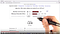Calculating the sample size given the power, significance level and the effect size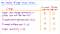How does the sample size change due to these changes?

## Analyze results

Finally, after the sample size is computed, we collect two groups of users and perform A/B testing. Specifically, compute CTP for each group, the difference, the 95% confidence interval of the difference, and decide whether the new feature should be launched.

machine learning and data science

## More from Yue

machine learning and data science